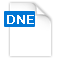3/5out of 5 based on 1 ratings. 1 user reviewsWhat is a dne file and how do I open a dne file?

`DNE is a file extension identified with Netica, a known Bayesian program that focuses on medicine, ecology, business and engineering.  Netica is an application developed by Norsys Software Corporation and deals with diagrams and belief networks.  Links between variables may be considered as probabilities which are expressed in equations or from established data.  Basically, any given case of which there is a limited idea, Netica will look for the appropriate answers and values for variables that are considered as unknown.  This in turn is expressed in meters and bar graphs which allows the user to analyze and deduce.  Netica is also capable of expressing a network in various ways.  Variables that don’t generate interest can be deducted without affecting the result of the variables.  The term Bayesian is referred to an 18th century theologian and mathematician named Thomas Bayes.  He gave the first mathematical treatment of a nontrivial problem of Bayesian inference which was later on continued by another French mathematician named Pierre Simon Laplace which is now known as Bayesian probability.          `
Windows